# TypeError: 'dict_keys' object is not subscriptable (Python)Last updated: Jan 31, 2023
7 min## #TypeError: 'dict_keys' object is not subscriptable (Python)

The Python "TypeError: 'dict_keys' object is not subscriptable" occurs when we try to access a dict_keys object at a specific index.

To solve the error, convert the `dict_keys` object to a list, before accessing an index, e.g. `list(my_dict.keys())`.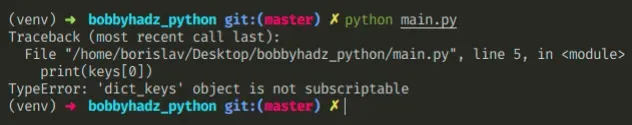Here is an example of how the error occurs.

main.py
```Copied!```my_dict = {'name': 'Bobby Hadz', 'age': 30}

keys = my_dict.keys()

print(type(keys))  # 👉️ <class 'dict_keys'>

# ⛔️ TypeError: 'dict_keys' object is not subscriptable
print(keys)
``````

Notice that the `dict.keys()` method returns a `dict_keys` object, not a `list`.

## #Convert the `dict_keys` object to a list

To solve the error, pass the `dict_keys` object to the `list` constructor to convert it to a list before accessing a list item at a specific index.

main.py
```Copied!```my_dict = {'name': 'Bobby Hadz', 'age': 30}

# ✅ convert to list
keys = list(my_dict.keys())

print(keys)  # 👉️ "name"
print(keys)  # 👉️ "age"
print(keys[0:2])  # 👉️ ['name', 'age']
``````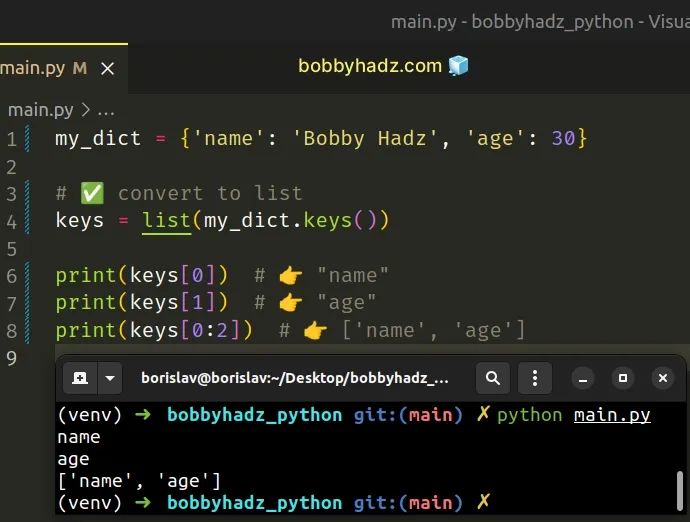The dict.keys method returns a new view of the dictionary's keys.

Since `dict_keys` objects are not subscriptable, we can't access them at a specific index.

Once you convert the `dict_keys` object to a list, you can also use list slicing.

main.py
```Copied!```my_dict = {'name': 'Bobby Hadz', 'age': 30, 'country': 'Belgium'}

keys = list(my_dict.keys())

print(keys[0:2]) # 👉️ ['name', 'age']
print(keys[1:3]) # 👉️ ['age', 'country']
``````

The syntax for list slicing is `a_list[start:stop:step]`.

The `start` index is inclusive and the `stop` index is exclusive (up to, but not including).

If the `start` index is omitted, it is considered to be `0`, if the `stop` index is omitted, the slice goes to the end of the list.

Python indexes are zero-based, so the first item in a list has an index of `0`, and the last item has an index of `-1` or `len(a_list) - 1`.

## #TypeError: 'dict_values' or 'dict_items' object is not subscriptable

The solution is the same if you got one of the following errors:

• TypeError: 'dict_values' object is not subscriptable
• TypeError: 'dict_items' object is not subscriptable

You have to convert the `dict_values` or `dict_items` object to a list before accessing it at an index.

Here is an example that does that for `dict_values`.

main.p
```Copied!```my_dict = {'name': 'Bobby Hadz', 'age': 30}

# ✅ convert to list
values = list(my_dict.values())

print(values)  # 👉️ 30

print(values[0:2])  # 👉️ ['Bobby Hadz', 30]
``````

And here is an example that does that for `dict_items`.

main.py
```Copied!```my_dict = {'name': 'Bobby Hadz', 'age': 30}

# ✅ convert to list
items = list(my_dict.items())

print(items)  # 👉️ ('name', 'Bobby Hadz')
print(items)  # 👉️ ('age', 30)

print(items[0:2])  # 👉️ [('name', 'Bobby Hadz'), ('age', 30)]
``````

## #Iterating over a dictionary's keys

You can iterate over a dictionary's keys without having to convert the `dict_keys` object to a list.

main.py
```Copied!```my_dict = {'name': 'Bobby Hadz', 'age': 30, 'country': 'Belgium'}

for key in my_dict.keys():
# name
# age
# country
print(key)
``````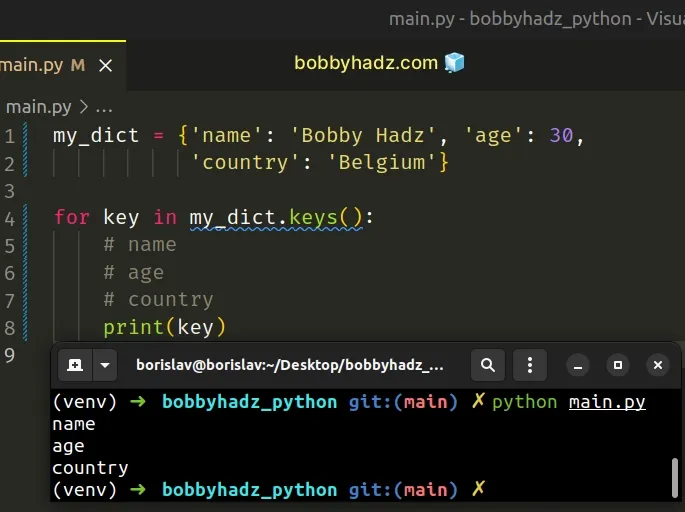On each iteration, we get access to the current key and print the result.

If you need to access the value of the current iteration, use the `dict.items()` method instead.

main.py
```Copied!```my_dict = {'name': 'Bobby Hadz', 'age': 30, 'country': 'Belgium'}

for key, value in my_dict.items():
# age 30
# country Belgium
print(key, value)
``````

## #Handling out-of-range exceptions with a `try/except`

Note that if you try to access a list index that is out of bounds, you would get an error.

You can use a try/except statement if you need to handle that.

main.py
```Copied!```my_dict = {'name': 'Bobby Hadz', 'age': 30}

keys = list(my_dict.keys())

try:
print(keys)
except IndexError:
print('index out of bounds')  # 👉️ this runs
``````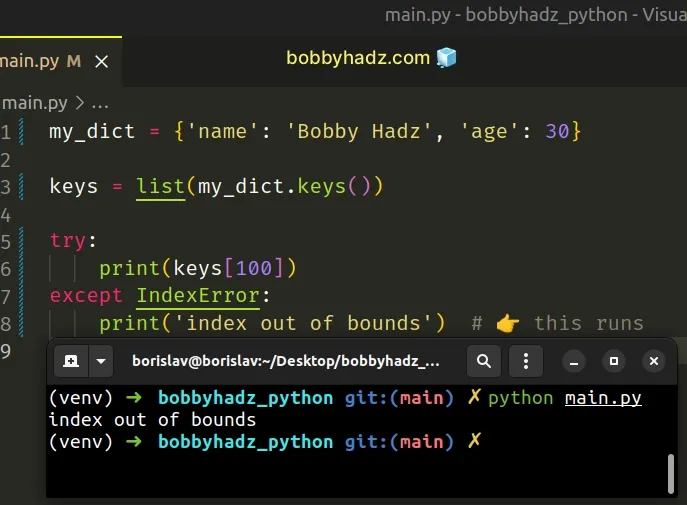The example catches the `IndexError` that is thrown if the index is out of bounds.

Note that an `IndexError` is not raised when you use list slicing, even if the specified indices do not exist.

main.py
```Copied!```my_dict = {'name': 'Bobby Hadz', 'age': 30, 'country': 'Belgium'}

keys = list(my_dict.keys())

print(keys[100:200])  # 👉️ []
print(keys[1:100])  # 👉️ ['age', 'country']
``````

The list constructor takes an iterable, such as a `dict_keys` object, and converts it to a list.

The `list` constructor builds a list whose items are the same and in the same order as the iterable's items.

The `list` constructor takes an iterable that may be a sequence, a container that supports iteration or an iterator object.

You should only use square brackets to access subscriptable objects.

The subscriptable objects in Python are:

• list
• tuple
• dictionary
• string

All other objects have to be converted to a subscriptable object by using the `list()`, tuple(), `dict()` or str() classes to be able to use bracket notation.

Subscriptable objects implement the `__getitem__` method whereas non-subscriptable objects do not.

main.py
```Copied!```a_list = [2, 4, 6, 8]

# 👇️ <built-in method __getitem__ of list object at 0x7f71f3252640>
print(a_list.__getitem__)
``````

## #TypeError: 'generator' object is not subscriptable in Python

The Python "TypeError: 'generator' object is not subscriptable" occurs when we try to access a generator object at a specific index.

To solve the error, pass the generator to the list constructor, e.g. `list(gen)`.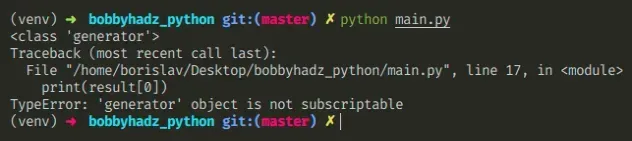Here is an example of how the error occurs

main.py
```Copied!```def g():
yield 1

yield 2

yield 3

gen = g()

print(type(gen))

# ⛔️ TypeError: 'generator' object is not subscriptable
print(gen)
``````

We used square brackets to try to access the element at index `0`, but the `generator` object is not subscriptable.

We can get around this by converting the `generator` object to a list.

main.py
```Copied!```def g():
yield 1

yield 2

yield 3

# ✅ convert generator object to list
my_list = list(g())

print(my_list)  # 👉️ [1, 2, 3]

print(my_list)  # 👉️ 1
print(my_list)  # 👉️ 2
``````

List objects are subscriptable, so we can access the element at a specific index after converting the `generator` object to a list.

You can iterate over a generator with a simple for loop.

main.py
```Copied!```def g():
yield 1

yield 2

yield 3

gen = g()

for i in gen:
print(i)  # 👉️ 1, 2, 3
``````

Alternatively, you can pass the `generator` to the `next()` function to retrieve the next item.

main.py
```Copied!```def g():
yield 1

yield 2

yield 3

gen = g()

print(next(gen))  # 👉️ 1
print(next(gen))  # 👉️ 2
print(next(gen))  # 👉️ 3
``````

Note that if you try to access a list index that is out of bounds, you would get an error. You can use a `try/except` statement if you need to handle that.

main.py
```Copied!```def g():
yield 1

yield 2

yield 3

my_list = list(g())

try:
print(my_list)
except IndexError:
print('index out of bounds')  # 👉️ this runs
``````

The example catches the `IndexError` that is thrown if the index is out of bounds.

The list constructor takes an iterable, such as a `generator` object, and converts it to a list.

The `list` constructor builds a list whose items are the same and in the same order as the iterable's items.

The `list` constructor takes an iterable that may be a sequence, a container that supports iteration or an iterator object.

You should only use square brackets to access subscriptable objects.

The subscriptable objects in Python are:

• list
• tuple
• dictionary
• string

All other objects have to be converted to a subscriptable object by using the `list()`, `tuple()`, `dict()` or `str()` classes to be able to use bracket notation.

Subscriptable objects implement the `__getitem__` method whereas non-subscriptable objects do not.

main.py
```Copied!```a_list = ['bobby', 'hadz', 'com']

# 👇️ <built-in method __getitem__ of list object at 0x7f71f3252640>
print(a_list.__getitem__)
``````

## #TypeError: 'map' object is not subscriptable in Python

The Python "TypeError: 'map' object is not subscriptable" occurs when we try to access a `map` object at a specific index.

To solve the error, convert the `map` object to a list before accessing it at an index, e.g. `new_list = list(map(int, a_list))`.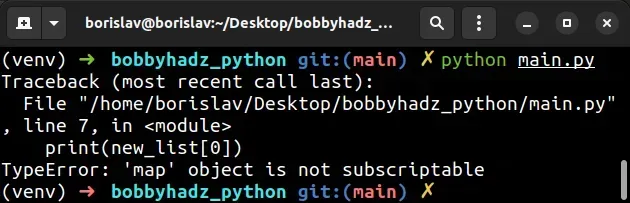Here is an example of how the error occurs.

main.py
```Copied!```a_list = ['2', '4', '6', '8']

new_list = map(int, a_list)
print(new_list)  # 👉️ <map object at 0x7fde832679a0>

# ⛔️ TypeError: 'map' object is not subscriptable
print(new_list)
``````

In Python 3, the `map()` function returns a `map` object, not a list.

To solve the error, use the `list()` class to convert the `map` object to a list.
main.py
```Copied!```a_list = ['2', '4', '6', '8']

new_list = list(map(int, a_list))
print(new_list)  # 👉️ [2, 4, 6, 8]

print(new_list)  # 👉️ 2
``````

The map() function takes a function and an iterable as arguments and calls the function with each item of the iterable.

The function returns a `map` object and `map` objects are not subscriptable (cannot be accessed at an index).

You can use square brackets if you need to access:

• a list at a specific index
• a tuple at a specific index
• a string at a specific index
• a specific key in a dictionary
An alternative approach to solving the error is to use a `for` loop to iterate over the `map` object without having to convert it to a list.
main.py
```Copied!```a_list = ['2', '4', '6', '8']

new_list = []

map_obj = map(int, a_list)

for item in map_obj:
print(int(item))

new_list.append(int(item))

print(new_list) # 👉️ [2, 4, 6, 8]

print(new_list)  # 👉️ 2

print(new_list[:2])  # 👉️ [2, 4]
``````

Map objects are evaluated lazily, so we used a `for` loop to iterate over the object.

You can optionally append the result of calling a function with each item to a list.

A better approach than having to convert a map object to a list is to use a list comprehension.

main.py
```Copied!```a_list = ['2', '4', '6', '8']

new_list = [int(item) for item in a_list]

print(new_list)  # 👉️ [2, 4, 6, 8]
``````

We used a list comprehension to iterate over the original list.

List comprehensions are used to perform some operation for every element or select a subset of elements that meet a condition.

On each iteration, we call the int() class with the current item and return the result.

The list comprehension returns a new list containing the results.

A list comprehension can be used as a replacement for the `map` function. All you have to do is call a function with each list item and return the result.

You can also use a list comprehension or the `map` function with user-defined functions.

main.py
```Copied!```def do_math(a):
return a + 100

a_list = [2, 4, 6, 8]

result = list(map(do_math, a_list))
print(result)  # 👉️ [102, 104, 106, 108]
print(result)  # 👉️ 102

result = [do_math(item) for item in a_list]
print(result)  # 👉️ [102, 104, 106, 108]
print(result)  # 👉️ 102
``````

The error basically means that the object cannot be accessed using square brackets.

You should only use square brackets to access subscriptable objects.

The subscriptable objects in Python are:

• list
• tuple
• dictionary
• string

All other objects have to be converted to a subscriptable object by using the `list()`, `tuple()`, `dict()` or `str()` classes to be able to use bracket notation.

Subscriptable objects implement the `__getitem__` method whereas non-subscriptable objects do not.

main.py
```Copied!```a_list = [1, 2, 3]

# 👇️ <built-in method __getitem__ of list object at 0x7f71f3252640>
print(a_list.__getitem__)
``````